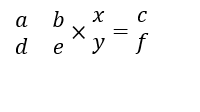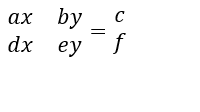# Write as a Matrix Worksheets

How to Write Linear Systems as a Linear Matrix? Equations are mathematical statements that include constants, variables, arithmetic operations, with an equals-to sign. There are different forms of the equation, and the most basic form is the linear equations. These equations have a variable with the highest power 1. The general form of a linear equation is given by;
ax + by = c
Here a, b, and c are constants, and x, y are variables. When there is a system of linear equations, we can also write it in the form of a linear matrix. What is a matrix? Well, a matrix is an arrangement of numbers in a rectangular form; it comprises of rows and columns. A linear matrix is one which represents a system of linear equations So, how to write linear systems as a linear matrix? Consider a linear system;
ax+by=c
dx+ey=f
To create a linear matrix, you need to create a co-efficient matrix, a variable matrix, and a constant matrix. A co-efficient matrix comprises of all the co-efficient of the variables. In this case, it becomes;
a  b
d  e
Now, the variable matrix can be written as; (x/y) The constant matrix is written as; (c/f) The system can now be written as;When you apply matrix multiplication here; it will become;• ### Basic Lesson

Guides students through writing linear equations as matrices. Step 1: Write the coefficients of x in the first column of first matrix. Step 2: Write the coefficients of y in the second column of first matrix. Step 3: Write x, y in the second matrix column wise. Step 4: Write the equivalent of the equations in the third matrix putting a "=" sign in between them.

• ### Intermediate Lesson

Demonstrates a step-by-step method for converting linear equations to matrices. Do not forget to write x,y,z in the second matrix column wise.

• ### Independent Practice 1

A really great activity for allowing students to understand the concept of Write as a Matrix.

• ### Independent Practice 2

Students find the Write as a Matrix in assorted problems. The answers can be found below.

• ### Homework Worksheet

Students are provided with problems to achieve the concepts of Write as a Matrix.

• ### Skill Quiz

This tests the students ability to evaluate Write as a Matrix.

• ### Homework and Quiz Answer Key

Answers for the homework and quiz.